GRE Subject Test: Chemistry : Endergonic and Exergonic Reactions

Example Questions

Example Question #15 : Thermodynamics And Phases

The equation for the change in Gibbs free energy is given below.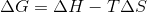ΔH = change in enthalpy

ΔS = change in entropy

Which of the following scenarios guarantees a nonspontaneous reaction?

When ΔH is negative and ΔS is positive

When ΔH is positive and ΔS is negative

When ΔH is negative and ΔS is negative

When ΔH is positive and ΔS is positive

When ΔH is positive and ΔS is negative

Explanation:

A positive value for ΔG (Gibbs free energy) will guarantee a nonspontaneous reaction. When ΔH (enthalpy) is postive and ΔS (entropy) is negative, the change in Gibbs free energy must be positive and, therefore, nonspontaneous.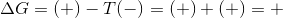Because T (temperature) will always have a positive value, a negative entropy and positive enthalpy will always result in a positive Gibbs free energy.

Example Question #16 : Thermodynamics And Phases

The equation for the change in Gibbs free energy is given below.ΔH = change in enthalpy

ΔS = change in entropy

In which of the scenarios will the reaction be spontaneous?

All of these scenarios would result in spontaneous reactions

When both ΔH and ΔS are negative and T is low

When both ΔH and ΔS are postive and T is high

When ΔH is negative and ΔS is postive

All of these scenarios would result in spontaneous reactions

Explanation:

All of the following scenarios would lead to spontaneous reaction, since each scenario would result in a negative Gibbs free energy (-ΔG).Negative enthalpy, positive entropy: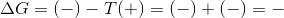Positive enthalpy and entropy with high temperature: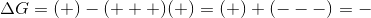Negative enthalpy and entropy with low temperature: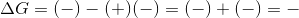Example Question #17 : Thermodynamics And Phases

Which of the following scenarios describes a reaction in equilibrium?

When Gibbs free energy is negative

When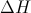is equal to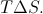When the enthalpy is equal to the entropy

When the forward rate is 0M/s

Whenis equal toAccording to the Gibbs free energy equation, a system is at equilibrium when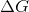is equal to 0.
Since, a system is in equilibrium when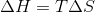. A negative Gibbs free energy means that the reaction will be spontaneous. At equilibrium, the forward reaction rate equals the reverse reaction rate, though the net rate is zero.